# RD Sharma Class 6 ex 19.6 Solutions Chapter 19 Geometrical Constructions

In this chapter, we provide RD Sharma Class 6 ex 19.6 Solutions Chapter 19 Geometrical Constructions for English medium students, Which will very helpful for every student in their exams. Students can download the latest RD Sharma Class 6 ex 19.6 Solutions Chapter 19 Geometrical Constructions Maths pdf, Now you will get step by step solution to each question.

# Chapter 19: Geometrical Constructions Exercise 19.6

### Question: 1

Construct an angle of 60° with the help of compasses and bisect it by paper folding.

### Solution: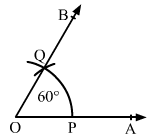Draw a ray OA.

With convenient radius and centre O, draw an arc cutting the ray OA at P.

With the same radius and centre at P, draw another arc cutting the previous arc at Q.

Draw OQ and extend it to B.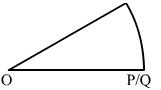∠AOB is the required angle of 60°.

We cut the part of paper as sector OPQ.

Now, fold the part of paper such that line segments OP and OQ get coincided.

Angle made at point O is the required angle, which is half of angle ∠AOB.

### Question: 2

Construct the following angles with the help of ruler and compasses only.

(i) 30°

(ii) 90°

(iii) 45°

(iv) 135°

(v) 150°

(vi) 105°

### Solution:

(i) 30°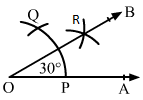Draw a ray OA.

With a convenient radius and centre at O, draw an arc, which cuts OA at P.

With the same radius and centre at P, draw an arc cutting the previous arc at P.

Taking P and Q as centres and radius more than half of PQ, draw two arcs, which cuts each other at R.

Draw OR and extend it to B.

∠AOB is the required angle of 30°.

(ii) 90°Draw a ray OA.

With a convenient radius and centre at O, draw an arc cutting the ray OA at P.

With the same radius and centre at P, draw another arc, which cuts the first arc at Q.

With the same radius and centre at Q, draw another arc, which cuts the first arc at R.

With Q and R as centres and radius more than half of QR, which cuts each other at S.

Draw OS and extend it to B from the ray OB.

∠AOB is required angle of 90°.

(iii) 45°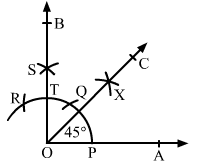To construct an angle of 45°, construct an angle of 900 and bisect it.

Construct the angle ∠AOB = 90°, where rays OA and OB intersect the arc at points P and T as shown in figure.

With P and T as centres and radius more than half of PT, draw two arcs, which cut each other at X

Draw OX and extend it to C to form the ray OC.

∠AOC is the required angle of 45°.

(iv) 135°

Draw the line AB and take the point O at the middle of AB.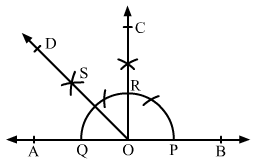With a convenient radius and centre at O, draw an arc, which cuts AB at P and Q, respectively.

Draw an angle of 90° on the ray OB as ∠BOC = 90°, where ray OC cuts the arc at R.

With Q and R as centres and radius more than half of QR, draw two arcs, which cuts each other at S.

Draw OS and extend it to form the ray OD.

∠BOD is required angle of 135°.

(v) 150°

Draw a line AB and take point O at the middle of AB.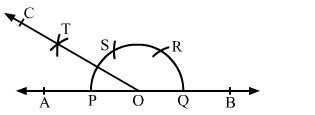With a convenient radius and centre at O, draw an arc, which cuts the line AB at P and Q.

With the same radius and centre at Q, draw an arc, which cuts the first arc at R.

With the same radius and centre at R, draw an arc, which cuts the first arc at S.

With the centres P and S and radius more than half of PS, draw two arcs, which cut each other at T.

Draw OT and extend it to C to form the ray OC.

∠BOC is required angle of 150°.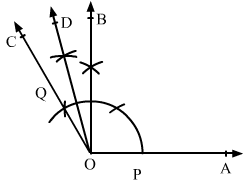(vi) 105°

Draw a ray OA and make an angle ∠AOB = 90° and ∠AOC = 120°

Now bisect ∠BOC and get the ray OD.

∠AOD is the required angle of 105°

### Question: 3

Construct a rectangle whose adjacent sides are 8 cm and 3 cm.

### Solution: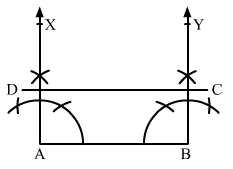Draw a line segment AB of length 8 cm.

Construct ∠BAX = 90° at point A and ∠ABY = 90° at point B.

Using a compass and ruler, mark a point D on the ray AX such that AD = 3 cm.

Similarly mark the point C on the ray Y such that BC = 3 cm.

Draw the line segment CD.

ABCD is the required rectangle.

All Chapter RD Sharma Solutions For Class 6 Maths

I think you got complete solutions for this chapter. If You have any queries regarding this chapter, please comment on the below section our subject teacher will answer you. We tried our best to give complete solutions so you got good